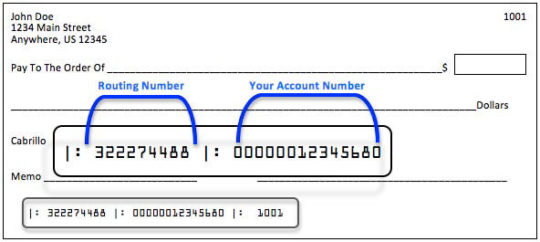# What is the correct formatting for my account number?

You can find the correctly formatted account number on the bottom of one of your Unitus checks. The first group of numbers on the left is the Unitus routing number. The next group of numbers is your account number.For direct deposit, you’ll need a 14-digit number. To convert your Unitus checking account number into a correctly formatted 14-digit number, add “1” to the beginning, then add zeros between the “1” and your account number, as needed, to reach 14 digits. Here is an example:

Example account number: 199240616
This account number is 9 digits long, so we’ll add 1 plus four zeros to the front of the number to reach 14 digits: 10000199240616

If you have questions, visit a branch or call our contact center at 503.227.5571 or 1.800.452.0900 to obtain the correctly formatted account number for direct deposit.

Main Phone Number

503.227.5571
1.800.452.0900

M-F 7:30a to 6:00p, Sat 7:30a to 4:00p

See all contact information